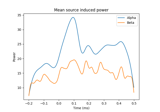# mne.minimum_norm.source_band_induced_power¶

mne.minimum_norm.source_band_induced_power(epochs, inverse_operator, bands, label=None, lambda2=0.1111111111111111, method='dSPM', nave=1, n_cycles=5, df=1, use_fft=False, decim=1, baseline=None, baseline_mode='logratio', pca=True, n_jobs=1, prepared=False, method_params=None, use_cps=True, verbose=None)[source]

Compute source space induced power in given frequency bands.

Parameters
epochsinstance of `Epochs`

The epochs.

inverse_operatorinstance of `InverseOperator`

The inverse operator.

bands`dict`

Example : bands = dict(alpha=[8, 9]).

label`Label`

Restricts the source estimates to a given label.

lambda2`float`

The regularization parameter of the minimum norm.

method“MNE” | “dSPM” | “sLORETA” | “eLORETA”

Use minimum norm, dSPM (default), sLORETA, or eLORETA.

nave`int`

The number of averages used to scale the noise covariance matrix.

n_cycles

Number of cycles. Fixed number or one per frequency.

df`float`

Delta frequency within bands.

use_fftbool

Do convolutions in time or frequency domain with FFT.

decim`int`

Temporal decimation factor.

baseline`None` (default) or `tuple`, shape (2,)

The time interval to apply baseline correction. If None do not apply it. If baseline is (a, b) the interval is between “a (s)” and “b (s)”. If a is None the beginning of the data is used and if b is None then b is set to the end of the interval. If baseline is equal to (None, None) all the time interval is used.

baseline_mode‘mean’ | ‘ratio’ | ‘logratio’ | ‘percent’ | ‘zscore’ | ‘zlogratio’

Perform baseline correction by

• subtracting the mean of baseline values (‘mean’)

• dividing by the mean of baseline values (‘ratio’)

• dividing by the mean of baseline values and taking the log (‘logratio’)

• subtracting the mean of baseline values followed by dividing by the mean of baseline values (‘percent’)

• subtracting the mean of baseline values and dividing by the standard deviation of baseline values (‘zscore’)

• dividing by the mean of baseline values, taking the log, and dividing by the standard deviation of log baseline values (‘zlogratio’)

pcabool

If True, the true dimension of data is estimated before running the time-frequency transforms. It reduces the computation times e.g. with a dataset that was maxfiltered (true dim is 64).

n_jobs`int`

The number of jobs to run in parallel (default 1). Requires the joblib package.

preparedbool

If True, do not call `prepare_inverse_operator()`.

method_params

Additional options for eLORETA. See Notes of `apply_inverse()`.

New in version 0.16.

use_cpsbool

Whether to use cortical patch statistics to define normal orientations for surfaces (default True).

Only used when the inverse is free orientation (`loose=1.`), not in surface orientation, and `pick_ori='normal'`.

New in version 0.20.

verbose

If not None, override default verbose level (see `mne.verbose()` and Logging documentation for more). If used, it should be passed as a keyword-argument only.

Returns
stcs

The estimated source space induced power estimates.

## Examples using `mne.minimum_norm.source_band_induced_power`¶